SOLVE IT!

If you live long enough (and well enough!), then eventually you are going to have to solve for x. It's unavoidable. Get used to it. Below are a variety of examples involving both symbolic and numerical solutions of equations and systems of equations. Enjoy!

Here are the functions and expressions.

> restart;

> y1:=2*x+5;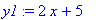> y2:=-x+10;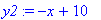> y3:=x^3-x^2+x-2;> f:=x->x^2-x-1;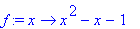> g:=x^2+y^2;Here are the solutions.

> solve(y1=0, x);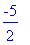> solve({y=y1,y=y2},{x,y});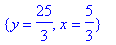> p:=solve(y3=0, x);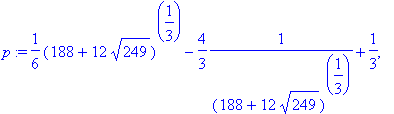> p;> evalf(p);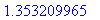> solve(f(x)=0, x);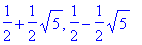> solve(g=1, y);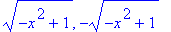And now, here are the floating point solutions (more or less).

> fsolve(y1=0, x);> fsolve({y=y1,y=y2},{x,y});> fsolve(y3=0, x);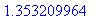> fsolve(y3=0, x, complex);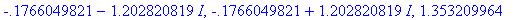> fsolve(y3=0, x, 0..2);> fsolve(f(x)=0, x);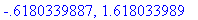>